## Sunday, September 13, 2020

### Infinite plane rendering 3: Image texturing, filtering, and antialiasing

This post is part three on the topic of infinite plane rendering. See part 1 and part 2.

Last time we textured the plane with a simple procedural texture. Now we add a proper image texture. First we use the texture coordinates from the procedural texture in part 2, to place a repeated version of a proper image on our infinite plane.

### Filtering

Without texture filtering, we see many artifacts. Notice the graininess toward the horizon. Also note the Moire patterns in the patterns of parallel lines at near to mid distance: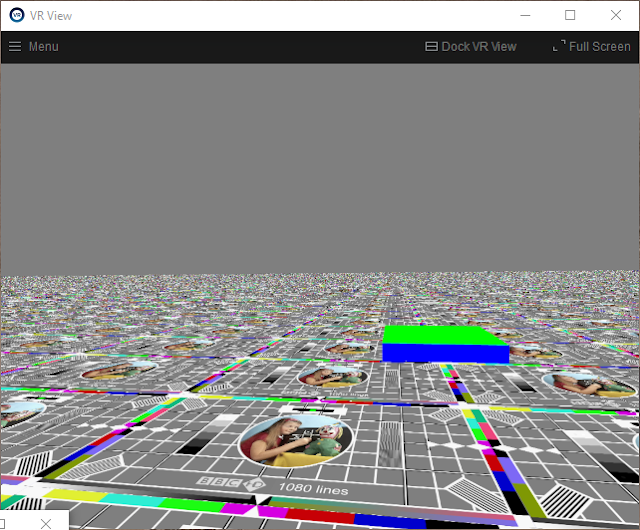Inifinite plane tiled with an unfiltered image texture. The image looks sorta OK up close, but everything is grainy at larger distances, and patterns of fine lines look bad even at moderate distances.

We can clear up most of those artifacts with trilinear filtering. When creating the texture, call
    glGenerateMipmap(GL_TEXTURE_2D);
and
    glTexParameteri(GL_TEXTURE_2D, GL_TEXTURE_MIN_FILTER, GL_LINEAR_MIPMAP_LINEAR);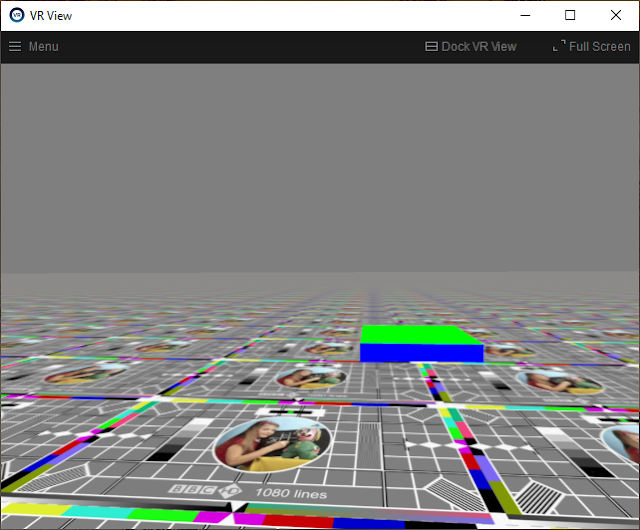Compared to the earlier unfiltered image, this trilinear filtered view avoids most of the artifacts. But the areas near the horizon get too blurry too fast.

Regular texture filtering does not work perfectly for oblique viewpoints, which is always the case near the horizon for our infinite plane. We need to use a technique called anisotropic filtering, which applies different filtering in different directions. Anisotropic filtering is not supported in pure OpenGL, so you need to load an extension GL_TEXTURE_MAX_ANISOTROPY_EXT and use it like this.
   glGetFloatv(GL_MAX_TEXTURE_MAX_ANISOTROPY_EXT, &f_largest);
   glTexParameterf(GL_TEXTURE_2D, GL_TEXTURE_MAX_ANISOTROPY_EXT, f_largest);

With anisotropic filtering, the texture pattern looks better near the horizon (below):Anisotropic filtering looks better by increasing the sharpness near the horizon. There remain some minor artifacts and shimmering, especially when viewed in VR. But this is the best I know how to do. Besides, we would ordinarily use much less demanding texture images. This texture is specifically designed to reveal display imperfections.

Using a more natural texture image, like the patch of grass below, shows no artifacts when viewed in VR using anisotropic filtering:

After gazing at this infinite vista of grass in VR, the game "Infinimower" practically writes itself.

### Efficiency and Antialiasing

Up to this point we have been inefficiently drawing the plane. The vertex shader creates a procedural triangle strip covering the entire screen, and the fragment shader rejects all the pixels not on the infinite plane using the "discard" command. So even if you are looking up, away from the plane, the fragment shader is still executed for every single pixel on the screen. We can be more efficient than this, and also solve the problem of antialiasing at the horizon line at the same time.So far we have been using a procedural vertex buffer, defined in the vertex shader, that covers the entire visible screen (pink rectangle). This is inefficient because the fragment shader gets invoked for each sky pixel too, even though these are never displayed.Here we use a more carefully selected vertex set, that matches the exact visible area of the infinite plane. This is a more efficient imposter geometry.

It would be possible to compute the optimal vertices on the host side, but today we'll compute it using a geometry shader program.

This also solves the horizon aliasing problem. Now that we have a triangle boundary along the horizon, it can be antialiased using multisample antialiasing (MSAA). This is a widely used technique so I won't describe the details here.

Shader source code is at https://gist.github.com/cmbruns/5b1c2b211766cdcb3b29689e0c32a63d

### Next Time:

• Using a 360 photo to cover the entire plane.

## Wednesday, May 13, 2020

### Infinite Plane Rendering 2: Texturing and depth buffer

This is the second post about rendering infinite planes in 3D computer graphics. Last time we got as far as rendering a uniform brown plane. This time we will add texturing and correct use of the Z/depth buffer."Brown plane" example from the previous post.

### Simplifying the math

Now that I'm looking at all this again, I see that we can simplify the plane math somewhat. Sorry I'm now going to change the variable names to match the plane ray tracing derivation in the OpenGL Super Bible. By the way, we aren't actually doing ray tracing in this infinite plane project, but rather simply "ray casting".

Remember the implicit plane equation from last time:

Ax + By + Cz + Dw = 0  (1b)

Where:

• (A B C) is a vector normal to the plane,
• (x y z) is any point in the plane, and
• D is the signed distance from the plane to the origin

We can rewrite that in vector form:

P·N + d = 0    (1c)

Where:

• N is a vector perpendicular to the plane
• P is any point on the plane, and
• d is the signed distance of the plane from the origin
That dot represents the dot product or inner product of two vectors.

We will combine the plane equation above with the ray equation below:

P = O + tD    (3)

Where:

• P is any point along the ray,
• O is the origin of the ray (i.e. the camera/viewer location),
• D is the direction of the view ray, and
• t is the scalar ray parameter.
Plug (3) into (1c) to get:

(O + tD)·N + d = 0

now solve for t:

t = -(O·N + d)/(D·N)

and plug that back into the ray equation to get I, the intersection between the plane and the view ray:

I = O - D(O·N + d)/(D·N)

Now we can simplify this further: If we solve for the intersection of the plane and the view ray in view/camera space, then the view ray origin O, the position of the camera/eye, is all zeros (0, 0, 0), and the intersection point equation reduces to:

I = -dD/(D·N)  (4)

That's pretty simple.

In our vertex shader, we compute the intersection point and pass it into the fragment shader as a homogeneous coordinate, with the denominator in the homogeneous w coordinate, as we discussed last time. A GLSL vertex shader code fragment is shown below:

point = vec4(
-d * D.xyz,  // xyz, numerator
dot(D.xyz, N.xyz)  // w, denominator

);

### Texturing the plane

That solid brown plane from the previous post could be hiding all sorts of errors. By adding texturing to the plane, we can visually distinguish different parts of the plane, so it becomes much easier to carefully verify correct rendering from inside the VR headset.

There are 4 different types of texturing we could apply to our plane:

1. Solid color rendering, like we did in the previous brown plane example.
2. Ordinary 2D image texture rendering, where an image pattern is repeated over the plane.
3. Procedural texture rendering, where a computed texture is applied in the fragment shader.
4. Spherical image texture rendering, using a 360 image. This way we can paint the whole plane with one single image. That's a great way to combine the special "whole universe" coverage of spherical panoramas, with the "half universe" coverage of infinite planes. We will get to this final case in a future post.
For now we will start with the simplest of procedural textures: a color version of the texture coordinates themselves. This helps us to debug texturing, and establishes the basis for other more advanced texturing schemes.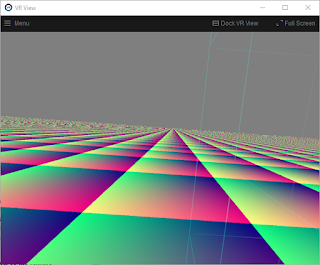A simple procedural texture tiling this infinite plane.
Now with the texturing in place, we can inspect the plane more carefully in VR, to help debug any rendering defects. For now it's (mostly) looking pretty good.

The grainy junk near the horizon is there because we have no texture filtering. Texture filtering for procedural textures like this is an advanced and difficult task. We won't be solving it here, because this is just a waypoint for us on the way to ordinary image-based texturing. There, the filtering techniques available to us will become much more conventional and straightforward.

### Populating the depth buffer

I mentioned in the previous post that our initial brown plane example does not correctly clip through other objects. Let's correct that now.

We can compute the correct depth buffer value for the plane intersection point by first converting the plane/ray intersection point into clip space (Normalized Device Coordinates) and then insert the depth value into the Z-buffer in the fragment shader.

vec4 ndc = projection * point;
...
gl_FragDepth = (ndc.z / ndc.w + 1.0) / 2.0;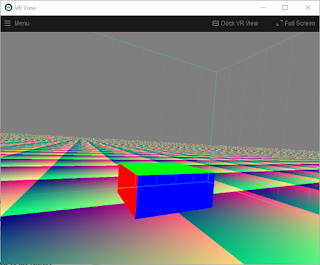Notice the top section of a cube mesh being correctly clipped by the infinite plane. The rest of the cube is correctly hidden beneath the surface of the plane. Thanks to gl_FragDepth.
There is one more nuance to using infinite planes with the depth buffer. We need to use a special sort of projection matrix that goes all the way to infinity, i.e. it has no far clip plane. So, for example, two infinite planes at different angles would clip each other correctly all the way to the horizon. To infinity! (but not beyond...)

Shader code for this version is at https://gist.github.com/cmbruns/3c184d303e665ee2e987e4c1c2fe4b56

### Topics for future posts:

• Image-based texturing
• Antialiasing
• Drawing the horizon line
• What happens if I look underneath that plane?
• More efficient imposter geometries for rendering

## Sunday, May 10, 2020

### How to draw infinite planes in computer graphics

Back in 2016 I figured out how to draw an infinite plane, stretching to the horizon, in my VR headset.  I did this because I wanted a very simple scene, with the ground at my feet, stretching out to infinity. This blog post describes some of the techniques I use to render infinite planes.

These techniques are done at the low-level OpenGL (or DirectX) layer. So you won't be doing this in Unity or Unreal or Blender or Maya. Unless you are developing or writing plugins for those systems.

The good news is that we can use the usual OpenGL things like model, view, and projection matrices to render infinite planes. Also we can use clipping, depth buffering, texturing, and antialiasing. But we first need to understand some maths beyond what is usually required for the conventional "render this pile of  triangles" approach to 3D graphics rendering. The infinite plane is just one among several special graphics primitives I am interested in designing custom renderers for, including infinite lines, transparent volumes, and perfect spheres, cylinders, and other quadric surfaces.

### Why not just draw a really big rectangle?

The first thing I tried was to just draw a very very large rectangle, made of two triangles. But the horizon did not look uniform. I suppose one could fake it better with a more complex set of triangles. But I knew it might be possible to do something clever to render a mathematically perfect infinite plane. A plane that really extends endlessly all the way to the horizon.

### How do you draw the horizon?

The horizon of an infinite plane always appears as a mathematically perfect straight line in the perspective projections ordinarily used in 3D graphics and VR. This horizon line separates the universe into two equal halves. Therefore, our first simple rendering of an infinite plane just needs to find out where that line is, and draw it.The ocean surface is not really an infinite plane, of course. But it is similar enough to help motivate our expectations of what a truly infinite plane should look like. Notice that the horizon is a straight line. Image courtesy of https://en.wikipedia.org/wiki/File:Into_the_Horizon.jpg
But before we figure out where that line is, we need to set up some mathematical background.

### Implicit equation of a plane

A particular plane can be defined using four values A, B, C, D in the implicit equation for a plane:

Ax + By + Cz + D = 0  (1a)

All points (x y z) that satisfy this equation are on the plane. The first three plane values, (A B C), form a vector perpendicular to the plane. I usually scale these three (A B C) values to represent a unit vector with length 1.0. Then the final value, D, is the signed closest distance between the plane and the world origin at (0, 0, 0).

This implicit equation for the plane will be used in our custom shaders that we are about to write. It's only four numbers, so it's much more compact than even the "giant rectangle" version of the plane, which takes at least 12 numbers to represent.

When rendering the plane, we will often want to know exactly where the user's view direction intersects the plane. But there is a problem here. If the plane is truly infinite, sometimes that intersection point will consist of obscenely large coordinate values if the viewer is looking near the horizon. How do we avoid numerical problems in that case? Good news! The answer is already built into OpenGL!

### Homogeneous coordinates to the rescue

In OpenGL we actually manipulate four dimensional vectors (x y z w) instead of three dimensional vectors (x y z). The w coordinate value is usually 1, and we usually ignore it if we can. It's there to make multiplication with the 4x4 matrices work correctly during projection and translation. In cases where the w is not 1, you can reconstruct the expected (x y z) values by dividing everything by w, to get the point (x/w, y/w, z/w, 1), which is equivalent to the point (x y z w).

But that homogeneous coordinate w is actually our savior here. We are going to pay close attention to w, and we are going to avoid the temptation to divide by w until it is absolutely necessary.

The general principle is this: if we see cases, like points near the plane horizon, where it seems like coordinate values (x y z) might blow up to infinity, let's instead try to keep the (x y z) values smaller and stable, while allowing the w value to approach zero. Allowing w to approach zero is mathematically equivalent to allowing (x y z) to approach infinity, but it does not cause the same numerical stability problems on real world computers - as long as you avoid dividing by w.

By the way, the homogeneous version of the plane equation is

Ax + By + Cz + Dw = 0  (1b)

### Intersection between view ray and plane

The intersection between a line and a plane (in vector notation, those are cross products and dot products below) is

I = ((Pn(VL)) - PV)/(Pn·V)    (2)

where I is the intersection point, Pn is the plane normal (A B C), V is the view direction, L is the camera/viewer location, and Pd is the D component of the plane equation.

Problems can occur when the denominator of this expression approaches zero. The intersection point needs to be expressed in the form (xyz)/w. Instead of setting w to 1, as usual, let's set w to the denominator Pn·V.

This means that the w (homogeneous) coordinate of the point on the plane intersecting a particular view ray will be the dot product of the view direction with the plane normal. The range of w values will range between -1 (when the view direction is directly opposite the plane normal) and +1 (when the view direction is exactly aligned with the plane normal). And the w value will be zero when the view is looking exactly at the horizon.

At this point, we can actually create our first simple rendering of an infinite plane. And we only need to consider the homogeneous w coordinate of the view/plane intersection point at first.

### Version 1: Brown plane

Here is the first version of our plane renderer, which only uses the homogeneous w coordinate: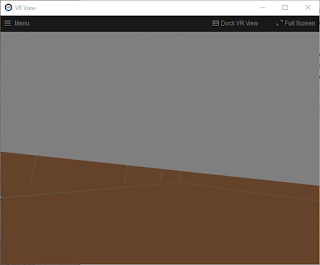Screenshot as I was viewing this infinite plane in VR

The horizon looks a little higher than it does when you look out at the ocean. But that's physically correct. The earth is not flat.

This plane doesn't intersect other geometry correctly, the horizon shows jaggedy aliasing, there's no texture, and it probably does not scale optimally. We will fix those things later. But this is a good start.

Source code for the GLSL shaders I used here is at at https://gist.github.com/cmbruns/815fc875afd8fe2755500907325b15f0

Continue to the next post in this series at https://biospud.blogspot.com/2020/05/infinite-plane-rendering-2-texturing.html

### Topics for future posts:

• Texturing the plane
• Using the depth buffer to intersect other geometry correctly
• Antialiasing
• Drawing the horizon line
• What happens if I look underneath that plane?
• More efficient imposter geometries for rendering